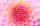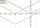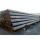Variable

Find variable P:

PP plus P x P plus P = 160

Result

P =  8

Solution:Checkout calculation with our calculator of quadratic equations.

Leave us a comment of example and its solution (i.e. if it is still somewhat unclear...):Be the first to comment!To solve this verbal math problem are needed these knowledge from mathematics:

Looking for help with calculating roots of a quadratic equation? Do you have a linear equation or system of equations and looking for its solution? Or do you have quadratic equation?

Next similar examples:

1. Evaluation of expressionsIf a2-3a+1=0, find (i)a2+1/a2 (ii) a3+1/a3
2. Square root 2If the square root of 3m2 +22 and -x = 0, and x=7, what is m?
3. Find twoFind two consecutive natural numbers whose product is 1 larger than their sum. Searched numbers expressed by a fraction whose numerator is the difference between these numbers and the denominator is their sum.Find the roots of the quadratic equation: 3x2-4x + (-4) = 0.
5. Variation equationSolve combinatorics equation: V(2, x+8)=72
6. Reciprocal equation 2Solve this equation: x + 5/x - 6 = 4/11
7. RootsDetermine the quadratic equation absolute coefficient q, that the equation has a real double root and the root x calculate: ?
8. Equation with abs valueHow many solutions has the equation ? in the real numbers?
9. DiscriminantDetermine the discriminant of the equation: ?
10. Solve 3Solve quadratic equation: (6n+1) (4n-1) = 3n2
11. EquationEquation ? has one root x1 = 8. Determine the coefficient b and the second root x2.Quadratic equation ? has roots x1 = 80 and x2 = 78. Calculate the coefficients b and c.Iron tubes in the warehouse are stored in layers so that each tube top layer fit into the gaps of the lower layer. How many layers are needed to deposit 100 tubes if top layer has 9 tubes? How many tubes are in bottom layer of tubes?If 5x + x² > 100, then x is notIn cinema are 1656 seats and in the last row are 105 seats , in each next row 3 seats less. How many are the total rows in cinema?On the trip went men, women and children in the ratio 2:3:5 by bus. Children pay 60 crowns and adults 150. How many women were on the bus when a bus was paid 4,200 crowns?We want to prove the sentence: If the natural number n is divisible by six, then n is divisible by three. From what assumption we started?